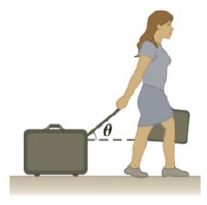Chapter 4, Problem 76AP

Chapter
Section
Textbook Problem

A woman at an airport is towing her 20.0-kg suitcase at constant speed by pulling on a strap at an angle θ above the horizontal (Fig. 4.76). She pulls on the strap with a 35.0-N force, and the friction force on the suitcase is 20.0 N. (a) Draw a free-body diagram of the suitcase. (b) What angle does the strap make with the horizontal? (c) What is the magnitude of the normal force that the ground exerts on the suitcase?Figure P4.76

(a)

To determine
The free body diagram.

Explanation

Given Info: The mass of the suitcase is 20 kg. Applied force is 35.0 N. Kinetic friction force is 20.0 N.

Explanation:

The free body diagram is given below.

• F is the applied force.
• m is the mass of the suitcase...

(b)

To determine
The angle between the strap and the horizon.

(c)

To determine
The magnitude of normal force.

Still sussing out bartleby?

Check out a sample textbook solution.

See a sample solution

The Solution to Your Study Problems

Bartleby provides explanations to thousands of textbook problems written by our experts, many with advanced degrees!

Get Started

Around the world, most people are lactose intolerant T F

Nutrition: Concepts and Controversies - Standalone book (MindTap Course List)

What are the moral implications of using ergogenic aids?

Understanding Nutrition (MindTap Course List)

How are granules and super granules related? How do they differ?

Horizons: Exploring the Universe (MindTap Course List)

2. In healthy men, the hematocrit is about

Cardiopulmonary Anatomy & Physiology